3.2.1.6 - Cell Calculations and AIM Triangle Flashcards Preview

MY biology > 3.2.1.6 - Cell Calculations and AIM Triangle > Flashcards

Flashcards in 3.2.1.6 - Cell Calculations and AIM Triangle Deck (18):
1

Identify what is missing from the unit conversion diagram.2

3

4

What can we use to help rearrange the equations for calculating image size, actual size and magnificaiton?5

Identify what is missing from the unit conversion diagram.6

7

8

9

Identify what is missing from the unit conversion diagram.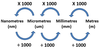10

11

Identify what is missing from the unit conversion diagram.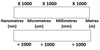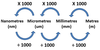12

13

14

15

Identify what is missing from the unit conversion diagram.16

Identify what is missing from the unit conversion diagram.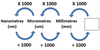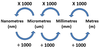17

18